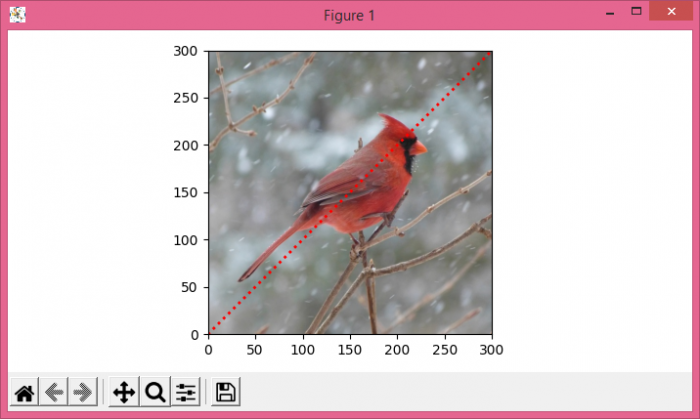# Matplotlib â€“ Plot over an image background in Python

To plot over an image background, we can take the following steps−

• Read an image from a file into an array.
• Create a figure (fig) and add a set of subplots (ax) with extent [0, 300, 0, 300].
• Create an array x of range (300).
• Plot x using plot() method with linestyle=dotted, linewidth=2, and color=red.
• To display the figure, use show() method.

## Example

import numpy as np
from matplotlib import pyplot as plt
plt.rcParams["figure.figsize"] = [7.00, 3.50]
plt.rcParams["figure.autolayout"] = True
plt.show()